# Josephson junction

(diff) ← Older revision | Latest revision (diff) | Newer revision → (diff)

Josephson received the 1973 Nobel Prize in Physics for discovery of the Josephson effect, which occurs in two superconducting layers separated by an insulating oxide. Under certain conditions current can pass through the insulator through tunneling of Cooper pairs of electrons. The effect has been used to design superconducting quantum interference devices (SQUID) because switching is very fast, in the order of picoseconds. Tunneling in the Josephson junctions is very sensitive to magnetic fields and can therefore be used to measure extremely small magnetic fields with thresholds as low as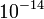Tesla. Josephson junctions are also used for other precision measurements. The standard volt is now defined as the voltage required to produce a frequency of 483,597.9 GHz in a Josephson junction oscillator.

A schematic diagram of a circuit with a Josephson junction is shown below.

The quantum effects can be modeled by the Schrodinger equation, but it turns out that the circuit can also be modeled as a system with lumped parameters. Let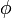be the flux that is the integral of the voltageacross the device,It follows from quantum theory [Feynman, 1970] that the current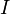through the device is a function of the flux: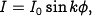where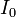is a device parameter, and the Josephson parameteris given by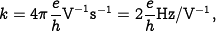where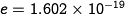Coluombs is the charge of an electron and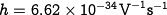is Planck's constant.

The circuit in the figure has two storage elements: the capacitor and the Josephson junction. We choose the states as the voltageacross the capacitor and the fluxof the Josephson junction. Let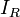,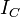and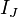be the currents through the resistor, the capacitor and the Josephson junction. We have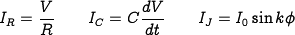and a current balance gives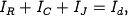which can be rewritten as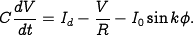Combining this equation with equation forgives the following state equation for the circuit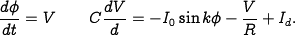Notice that apart from parameter values, this equation is identical to the equation for the inverted pendulum.